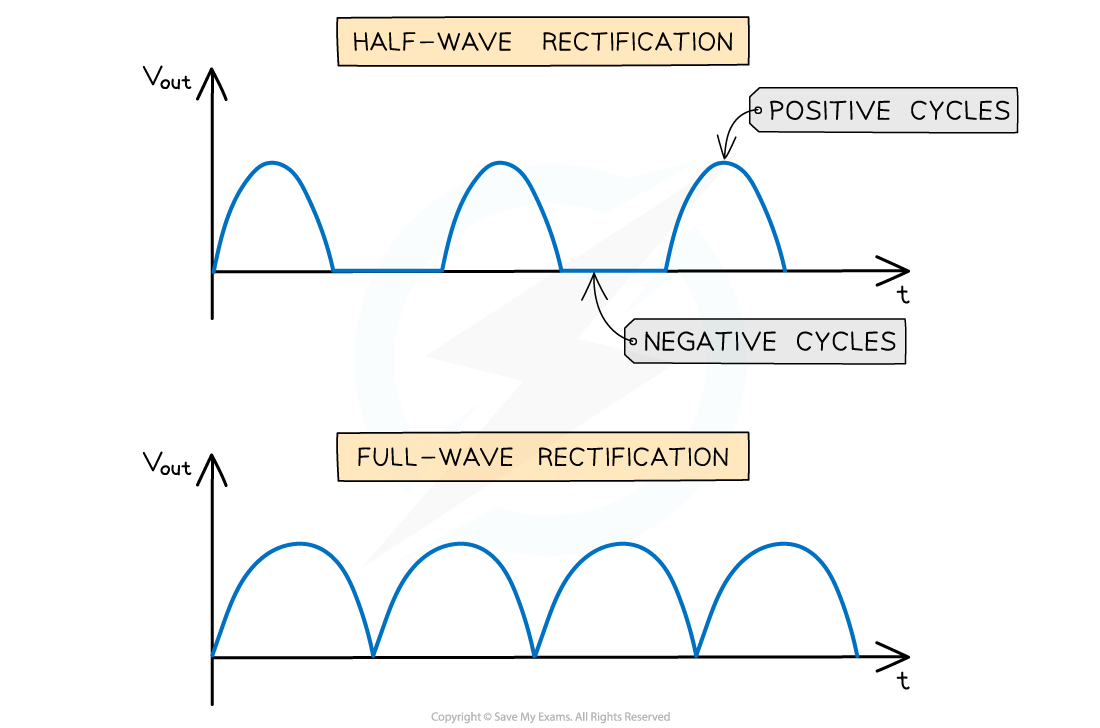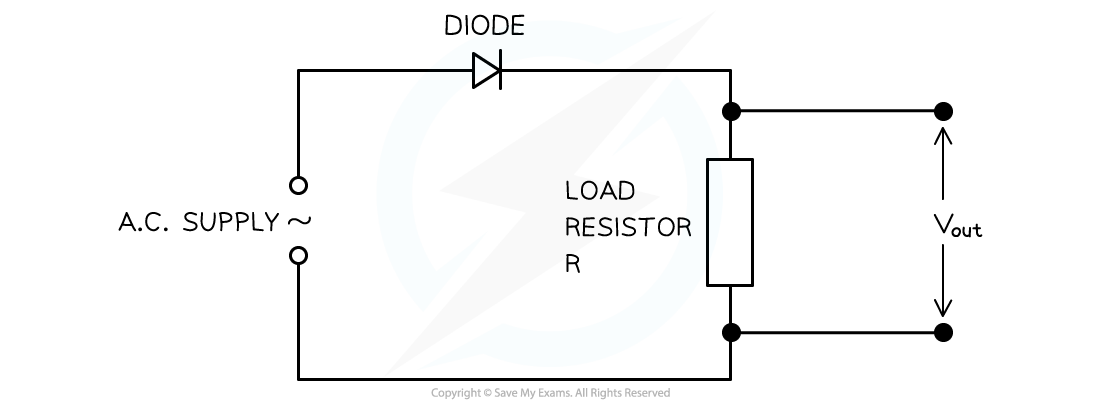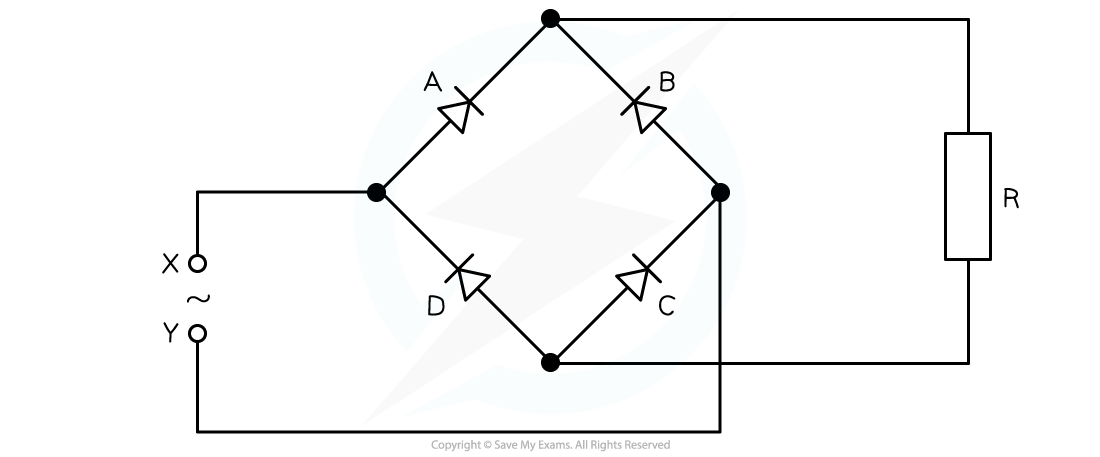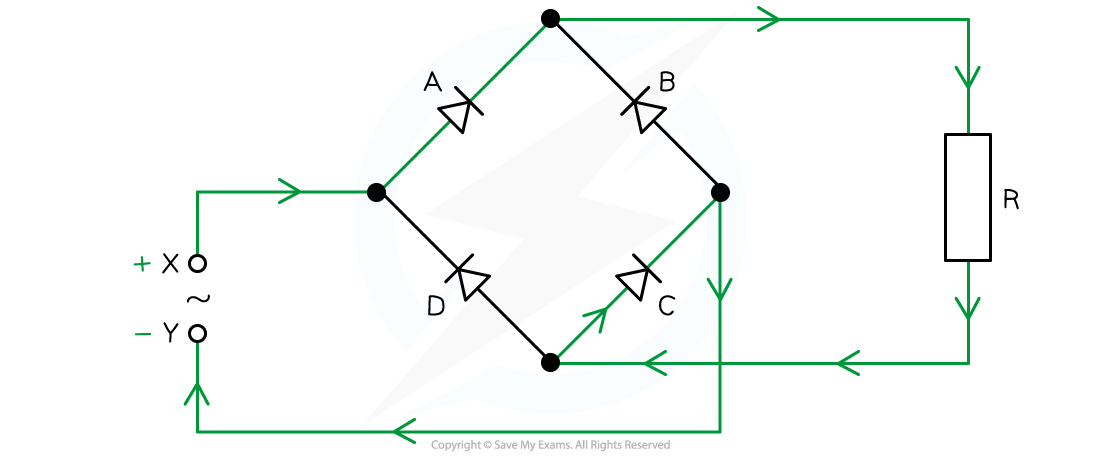# IB DP Physics: HL复习笔记11.2.8 Rectification

### Rectification

• Rectification is defined as:

The process of converting alternating current  and voltage into direct current  and voltage

• Rectification is used in electronic equipment which requires a direct current
• For example, mains voltage must be rectified from the alternating voltage produced at power stations
• There are two types of rectification:
• Half-wave rectification
• Full-wave rectification
• For half-wave rectification:
• The graph of the output voltage Vout against time is a sine curve with the positive cycles and a flat line (Vout = 0) on the negative cycle
• This is because the diode only conducts in the positive direction
• For full-wave rectification:
• The graph of the output voltage Vout against time is a sine curve where the positive cycles and the negative cycles are both curved ‘bumps’The difference between the graphs of full-wave and half-wave rectification

#### Half-Wave Rectification

• Half-wave rectification consists of a single diode
• An alternating input voltage is connected to a circuit with a load resistor and diode in series
• The diode will only conduct during the positive cycles of the input alternating voltage,
• Hence there is only current in the load resistor during these positive cycles
• The output voltage Vout across the resistor will fluctuate against time in the same way as the input alternating voltage except there are no negative cyclesHalf-wave rectification requires a single diode and the graph is represented by only the positive cycles

• This type of rectification means half of the time the voltage is zero
• The power available from a half-wave rectified supply is reduced

#### Full-Wave Rectification

• Full-wave rectification requires a bridge rectifier circuit
• This consists of four diodes connected across an input alternating voltage supply
• The output voltage Vout is taken across a load resistor
• During the positive cycles of the input voltage, one terminal if the voltage supply is positive and the other negative
• Two diodes opposite each other that are in forward bias will conduct
• The other two in reverse bias will not conduct
• A current will flow in the load resistor with the positive terminal at the top of the resistor
• During the negative cycles of the input voltage, the positive and negative terminals of the input alternating voltage supply will swap
• The two diodes that were in forward bias will now be in reverse bias and not conduct
• The other two in reverse bias will now be in forward bias and will conduct
• The current in the load resistor will still flow in the same direction as beforeWhen A is positive and B is negative, diodes 2 and 3 will conduct and 1 and 4 will not. When A is negative and B is positive, diodes 1 and 4 will conduct and diodes 2 and 3 will not. The current in the load resistor R will flow downwards

• In both the positive and negative cycles, the current in the load resistor is the same
• Each diode pair is the same as in half-wave rectification
• Since there are two pairs, this equates to full-wave rectification overall
• The main advantage of full-wave rectification compared to half-wave rectification is that there is more power available
• Therefore, greater power is supplied on every half cycle

#### Worked Example

A bridge rectifier consists of four ideal diodes A, B, C and D as connected in the figure shown below.An alternating supply is applied between the terminal X and Y.

Identify which diodes are conducting when terminal X of the supply is positive.

• Draw path of the current direction with diodes in forward bias
• Remember that conventional current flow is from positive to negative and only travels through the paths with diodes in forward bias• Therefore, the answer is: diodes A and C

#### Exam Tip

Being able to reproduce the diode bridge correctly and explain the cycles of full-wave rectification are important physics concepts that may occur during an examination. It is worth learning to draw and explain full-wave rectification accurately